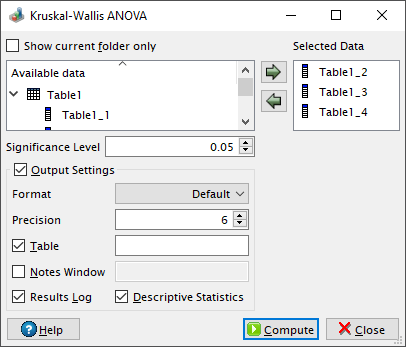# Kruskal-Wallis ANOVA Dialog

This dialog is activated by selecting the Kruskal-Wallis ANOVA... command from the Statistics -> Nonparametric Tests -> menu. It can be used in order to test whether several samples originate from the same distribution. QtiPlot uses the test procedure explained in the following Wikipedia article

Figure 5-104. The Kruskal-Wallis ANOVA dialog.QtiPlot computes the test statistic (H) and an approximated p-value. The approximation is based on the fact that when the size of the input samples (Ni) or the number of samples (k) is large (i.e. Ni > 5 or k ≥ 3), the probability distribution of H is that of a chi-squared distribution whith k-1 degrees of freedom (DoF). The p-value computed by QtiPlot is the probability of a chi-squared value of at least H being observed: 1-chi2cdf(H, k-1). The null hypothesis is rejected if the approximated probability is lower than the value of the significance level.

The data sets selected in the Available data window can be added to the analysis either by pressing the left to right arrow button or by dragging and dropping them onto the right side window.